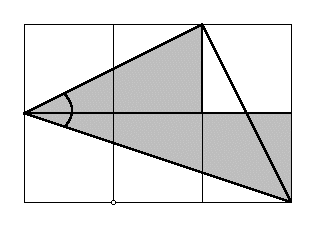Math 240                                                                M. Flashman
Proof without Words #2

The figure below contains information that can be used to explain why the following equation is true:

arctan (1/2) + arctan (1/3) = pi/4

Give a written explanation based on the figure for the truth of the equation.
[In your explanation be explicit in identifying what in the figure corresponds to arctan(1/2) and arctan(1/3).
What in the figure allows you to identify this sum with the number pi/4?]